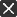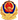• ###### 政民互动关闭
 索  引  号：111423LL00100/2021-01138 发文字号：吕政发〔2021〕13号 发布日期：2021年09月07日 发文机关：吕梁市人民政府 关  键  字： 标　　题：关于收回吕梁市商贸储运公司国有建设用地使用权的决定 主题分类：土地 成文日期：2021年09月07日

为实施《吕梁市中心城区货源街片区老旧小区改造工作方案》，依照《中华人民共和国土地管理法》《土地储备管理办法》和《吕梁市规划区土地储备实施办法》的规定，作出如下决定：

一、无偿收回你单位位于离石区货源街98号的国有建设用地使用权，面积为3668.45平方米。宗地四至为：东邻居民住宅、南邻市商务局家属院、西至小路、北至货源街。宗地坐标如下：

X1=4153811.3150， Y1=37511960.959；

X2=4153805.4430，         Y2=37511976.490；

X3=4153801.8910，         Y3=37511994.006；

X4=4153793.0100，         Y4=37512019.830；

X5=4153788.3090，         Y5=37512018.246；

X6=4153781.2840，         Y6=37512018.496；

X7=4153743.5190，         Y7=37512019.839；

X8=4153743.2120，         Y8=37512011.584；

X9=4153741.5760，         Y9=37511967.698；

X10=4153741.2000，         Y10=37511957.614；

X11=4153753.9320，         Y11=37511957.540；

X12=4153782.5600，         Y12=37511957.848；

X13=4153782.5610，         Y13=37511957.711；

X14=4153785.8570，         Y14=37511957.730；

X15=4153787.0220，         Y15=37511957.804；

X16=4153790.9060，         Y16=37511958.291；

X1=4153811.3150， Y1=37511960.959。

二、收回的国有建设用地由市土地储备中心储备管理，具体位置以《土地勘测定界技术报告书》编号：RX-(SC2021-003）号为准。

三、请你单位尽快到原土地登记机关办理国有建设用地的使用权注销登记。

2021年9月7日

（此件公开发布）

解读说明：此件不属于政策性文件，不作解读。

咨询电话：市规自局　土地储备中心　8296746晋公网安备 14110002000102号